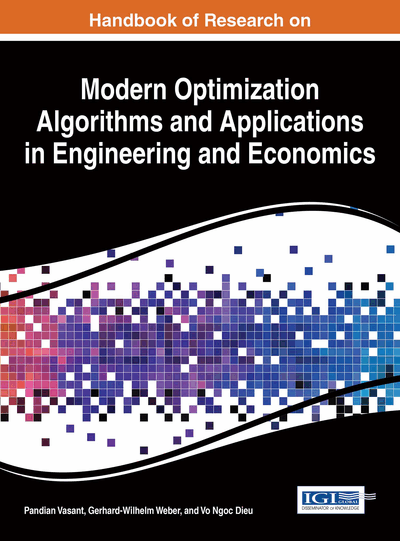# Variable Selection in Multiple Linear Regression Using a Genetic Algorithm

Javier Trejos (University of Costa Rica, Costa Rica), Mario A. Villalobos-Arias (University of Costa Rica, Costa Rica) and Jose Luis Espinoza (Technological Institute of Costa Rica, Costa Rica)
DOI: 10.4018/978-1-4666-9644-0.ch005

## Abstract

In this article it is studied the application of a genetic algorithm in the problem of variable selection for multiple linear regression, minimizing the least squares criterion. The algorithm is based on a chromosomic representation of variables that are considered in the least squares model. A binary chromosome indicates the presence (1) or absence (0) of a variable in the model. The fitness function is based on the adjusted square R, proportional to the fitness for chromosome selection in a roulette wheel model selection. Usual genetic operators, such as crossover and mutation are implemented. Comparisons are performed with benchmark data sets, obtaining satisfying and promising results.
Chapter Preview
Top

## Introduction

Model selection is a very important task in Statistics, as it can be viewed in several ways: a dimension reduction procedure, an important feature selection task. In any way, it simplifies the situation modeled and gives sense to the analysis of the data.ds

In multiple linear regression, a numerical variable Y is modeled by a linear combination of explanatory numerical variables. For minimizing the sum of squares of the differences between Y and this linear combination, it is well known (Draper & Smith (1968), Tomassone, Audrain, Lesquoy, & Millier (1992), Venables & Ripley (1994)) that a solution can be obtained if the Xj are not linearly dependent. In the case of collinearity between these variables, several approaches have been proposed for overcoming the problem: (i) Stepwise regression, selecting the most explanatory variables in a forward or backward greedy procedure; (ii) regularization via principal component analysis, using the independent principal components; (iii) use of metaheuristics for selecting the best explanatory subset of Xj.

There are many other types of regression. In recent years, the tree based algorithms have become very popular, such as CART (Breiman, Friedman, Ohlsen, & Stone, 1984; Gordini & Veglio, 2014). Also, fuzzy procedures are useful in some cases (de los Cobos, 2011). PLS regression is a generalization for the case of several variables to be explained. In the section of additional readings the authors have put several references to these and other situations.

Nonlinear regression is appropriate in cases where the linear model does not explain correctly the variable Y. The most well known method for non linear regression is the Gauss-Newton method, based on a first-order Taylor approximation, and iteratively approximating the solution. Another method is the gradient one, that looks for the steepest descent at each point. And the Marquardt method is some kind of combination of the preceding methods. Even if these approaches are reasonable, there is no guarantee of reaching the best least squares solution, moreover, in some cases the iterations may not converge at all. For this, somewhere else the authors have also applied metaheuristics with very good results in nonlinear regression, using simulated annealing and tabu search (Villalobos & Trejos, 2000; Villalobos, Trejos, & de los Cobos, 2006).

The problem of selecting explanatory variables in linear regression was tackled by using a genetic algorithm (Holland, 1975), a metaheuristic that has shown to behave properly in many difficult optimization methods (Vasant, 2013). For this, it is necessary to define an appropriate fitness function. In our case, a balance between two conflicting objectives is needed: (i) want to include all variables that have legitimate predicting skill; (ii) want to exclude any redundant or sample-specific variables. Of course, there is no single definition of “best”, and it is well known that different algorithms may produce different solutions and, in linear regression, problems are magnified by correlation among predictors.

Among the different criteria that may be used, in this article it is used one such that it increases only if new variables included add significantly to the model since it is not good to add too many explanatory variables that do not seem to contribute much to the model. Of course, this is not the case of the determination coefficient, since it increases with the number of variables in the model.

There are several criteria that may be used to tackle this problem: the Adjusted R square, the Mallow’s statistic, the Press statistic, the Akaike information criterion (AIC), the Bayesian information criterion (BIC), and so on. In this investigation, it was used the Adjusted R square, which can decline in value if the contribution to the explained deviation by the additional variable is less than the impact on the degrees of freedom. In the background section these criteria are developed in more detail.

## Key Terms in this Chapter

Genetic Algorithm: An iterative meta-heuristic based on the evolution of species, that handles a population of solutions of the optimization problem that have a survive probability proportional to the quality of the respective solution, and makes combinations of solutions based on crossover and mutation operators.

Variable Selection: Procedures that select the most explanatory variables in a regression model, according to some numerical criterion.

Metaheuristics: Optimization methods that search for good solutions of a problem, usually based on iterative steps that approximate the solution; the “meta” term refers to the fact that a basic frame is used to many different situations.

Regression: A set of methods that model one or more variables to be explained, usually numerical, by means of a set of explanatory variables, also usually numerical.

## Complete Chapter List

Search this Book:
Reset